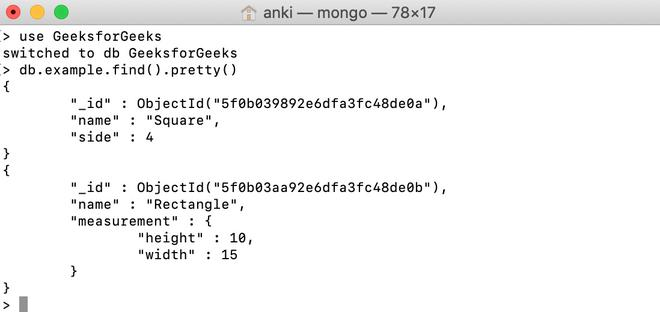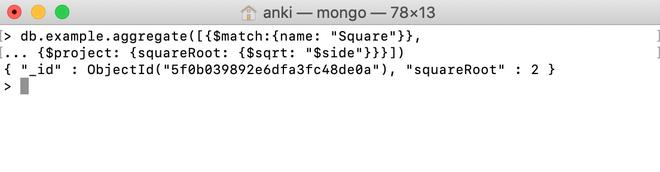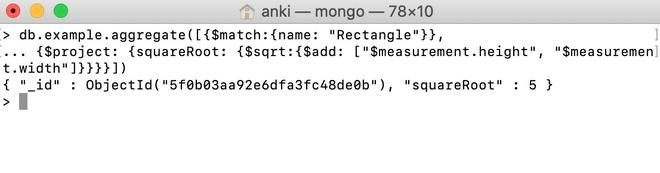# MongoDB \$sqrt Operator

MongoDB provides different types of arithmetic expression operators that are used in the aggregation pipeline stages \$sqrt operator is one of them. This operator is used to find the square root of a positive number and returns the result as a double.

Syntax:

```{ \$sqrt: <number> }
```

Here, the number is a valid expression until it resolves to a non-negative number.

• If the entered value is null, then this operator will return null.
• If the entered value is NaN, then this operator will return NaN.
• If the entered value is a missing field, then this operator will return null.

Examples:

In the following examples, we are working with:

Database: GeeksforGeeks

Collection: example

Document: two documents that contain the details of the shapes in the form of field-value pairs.Finding the square root of the field using \$sqrt operator:

In this example, we are going to find the square root of the value of the side field in the Square document using \$sqrt operator.

```db.example.aggregate([{\$match:{name: "Square"}},
... {\$project: {squareRoot: {\$sqrt: "\$side"}}}])

```Finding the square root of the field in the embedded document using \$sqrt operator:

In this example, we are going to find the square root of the sum of the values of the measurement.height and measurement.width fields in the Rectangle document using \$sqrt operator.

```db.example.aggregate([{\$match:{name: "Rectangle"}},